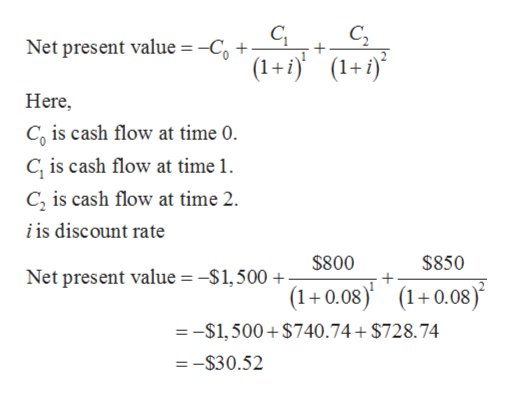# Find the net present value (NPV) and proﬁtability index (PI) of a project that costs \$1,500 and returns \$800 in year one and \$850 in year two. Assume the project’s cost of capital is 8 percent.

Question
6 views

Find the net present value (NPV) and proﬁtability index (PI) of a project that costs \$1,500 and returns \$800 in year one and \$850 in year two. Assume the project’s cost of capital is 8 percent.

check_circle

Step 1

The net present value for the project is calculated below:help_outlineImage TranscriptioncloseC2 (1+1(1+i) Net present value -Co + + Here, Co is cash flow at time 0. C is cash flow at time 1. C is cash flow at time 2 i is discount rate \$800 \$850 Net present value = -\$1,500 (1+0.08) (10.08) =-S1,500+\$740.74+ \$728.74 =-\$30.52 fullscreen
Step 2

The formula to calculate profitability index is given below:

Step 3

Substitute (\$740.74 + \$728.74) =\$1,469.48 for present value...

### Want to see the full answer?

See Solution

#### Want to see this answer and more?

Solutions are written by subject experts who are available 24/7. Questions are typically answered within 1 hour.*

See Solution
*Response times may vary by subject and question.
Tagged in